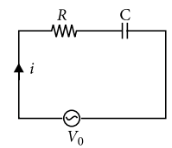Phasors and Phasor Diagrams
Question

# A series R-C circuit is connected to an alternating voltage source. Consider two situations:(a) When capacitor is air filled.(b) When capacitor is mica filled. Current through resistor is i and voltage across capacitor is V then

Moderate
Solution

##$=\frac{{V}_{0}}{\sqrt{{R}^{2}+{X}_{C}^{2}}}=\frac{{V}_{0}}{\sqrt{{R}^{2}+\left(1/\omega C{\right)}^{2}}}$$=\frac{{V}_{0}}{\sqrt{{R}^{2}+\left(1/\omega C{\right)}^{2}}}×\frac{1}{\omega C}=\frac{{V}_{0}}{\sqrt{{R}^{2}{\omega }^{2}{C}^{2}+1}}$

Get Instant Solutions